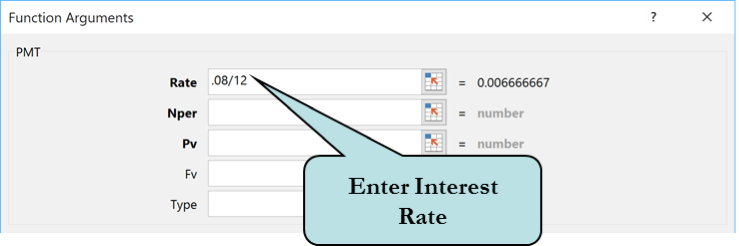# Calculate a Loan in Excel using the PMT Function

Posted by Roger Hyttinen on

The PMT function is used to calculate payments due on a loan based on constant payments and assuming a constant interest rate. With the PMT function, you can easily figure out what your monthly payments will be on a mortgage or car loan.

To calculate loan payments, the PMT function uses 3 arguments:

1. rate – the interest rate. If you had a loan that you had to pay monthly with an interest rate of 8%, this argument would read: .08/12 (interest rate divided by 12 months).
2. nper – the total number of payments of the loan. If the loan is a 30 year mortgage and you make a monthly payment, this argument would read: 12 * 30 (12 months times 30 years - or you can enter 360).
3. pv – the present value of the loan. This is the total loan amount upon which you want your formula to be based and is typically entered as a negative number. Thus, if your loan was for a \$150,000 mortgage, then this argument would read: -150000.

Thus, the format of the PMT function is:

=PMT(rate, nper, pv)

The PMT function can also be used to calculate an investment goal. For example, you can calculate how much money you need to invest in order to achieve a specific dollar amount, assuming a constant interest rate and number of payments. So rather than using the present value (pv) we need to enter the future value (fv).

The format of the PMT function to calculate an investment goal would be:

=PMT(rate, nper,, fv)

As an example, suppose you wanted to save \$15,000 in the next 5 years and you know that the interest rate for the account will be 7%. To calculate what your monthly investment would be, the formula would read:

=PMT(.07/12,12*5,,-15000)

Note: You can either type in the formula for the PMT function manually, or use the Insert Function button.

## Quick Guide: To Calculate a Loan with the PMT Function

1. Activate the cell in which you want to place the formula.
2. Click the Insert Function icon to the left of the toolbarOr
Click the Formulas tab and click the Insert Function button on the Function Library group (you can also click the Financial button on the Function Library and choose PMT from the list. Then, skip to step 6).
3. Select Financial from the Select a Category drop-down list.
4. From the Insert Function box, select PMT from the Select a Function list box.
5. Click OK.
6. Enter the interest rate per period (ie. Monthy: rate /12, Semiannual: rate /6, etc.) in the Rate text box.7. Enter the total number of payments of the loan in the Nper text box.
8. For loan payments, enter the loan amount as a negative number in the Pv text boxOr
For future value amount, enter the future value to be achieved as a negative number in the Fv text box.
9. Press the Enter key to verify the formula.